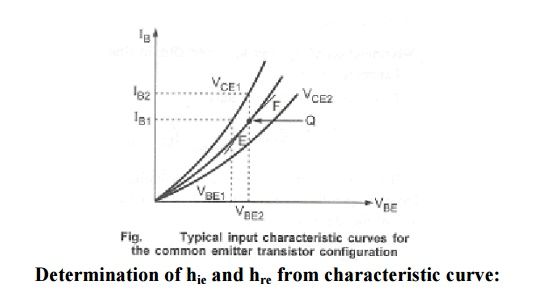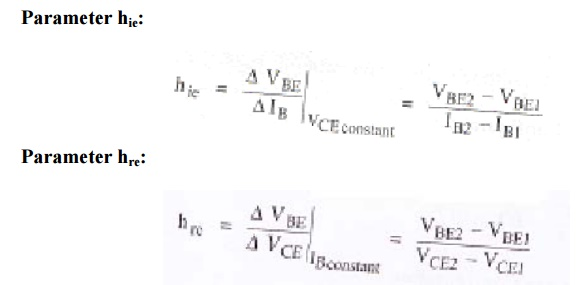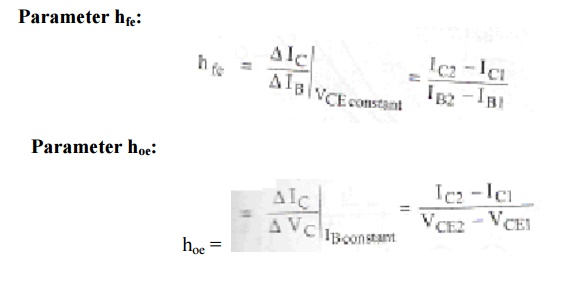Home | | Electronic Circuits I | h-Parameters for all three configurations

# h-Parameters for all three configurations

Transistor can be represented as two port network by making anyone terminal common between input and output.

h-Parameters for all three configurations:

Transistor can be represented as two port network by making anyone terminal common between input and output. There are three possible configurations in which a transistor can be used, there is a change in terminal voltage and current for different transistor configurations. To designate the type of configuration another subscript is added to h-parameters.

hie = h11e– input resistance in CE configuration

hfb = h21b – short circuit current gain in CB configuration

Table: Summarizes h-parameters for all three configurationsThe basic circuit of hybrid model is same for all three configurations, only parameters are different.The circuit and equations are valid for either NPN or PNP transistor and are independent of the type of load or method of biasing.

Determination of h-parameters from characteristics:

Consider CE configuration, its functional relationship can be defined from the following equations:The input characteristic curve gives the relationship between input voltage VBE and input current I B for different values of output voltage VCE. The following figure shows the typical input characteristic curve for CE configuration.The output characteristic curve gives the relationship between output current ICand output voltage VCE for different values of input current IB.Study Material, Lecturing Notes, Assignment, Reference, Wiki description explanation, brief detail
Electronic Circuits : BJT Amplifiers : h-Parameters for all three configurations |